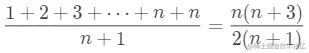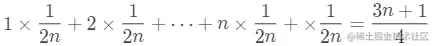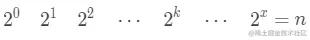# 最好、最坏、平均、均摊时间复杂度不同情况下，时间复杂度可能

《最好、最坏、平均、均摊时间复杂度》原文链接，阅读体验更佳

# 不同情况下，时间复杂度可能不同

## 最好、最坏情况时间复杂度

``````int find(int[] array, int value) {
if(array == null || array.length == 0) {
return -1;
}
for(int i = 0; i < array.length; i++) {
if(array[i] == value) {
return i;
}
}
return -1;
}

## 平均情况时间复杂度## 均摊时间复杂度

``````// array表示一个长度为n的数组
// 代码中的array.length就等于n
int[] array = new int[n];
int count = 0;

void insert(int val) {
if (count == array.length) {
int sum = 0;
for (int i = 0; i < array.length; ++i) {
sum = sum + array[i];
}
array = sum;
count = 1;
}

array[count] = val;
++count;
}

• 如果不出现数组中没有空闲位置这个特殊情况，代码的时间复杂度永远是O(1)，没有出现量级的差异；
• 数组中没有空闲位置这个特殊情况的出现具有一定的周期性，是在一个O(n)操作之后，紧跟着出现n-1个O(1)的插入操作，循环往复。

# 常见的时间复杂度

• 常量阶 O(1)

• 对数阶 O(logn)

• 线性阶 O(n)

• 线性对数阶 O(nlogn)

• 平方阶 O(n2)、立方阶 O(n3)...k次方阶 O(nk)

需要注意的是，这里的k次方阶中的k必须是一个常量，是与输入数据无关的

• 指数阶 O(2n)

• 阶乘阶 O(n!)

## O(1)

``````int i = 8;
int j = 6;
int sum = i + j;

## O(logn)、O(nlogn)

``````i=1;
while (i <= n)  {
i = i * 2;
}``````i=1;
while (i <= n)  {
i = i * 3;
}

## O(m+n)、O(m*n)

``````int cal(int m, int n) {
int sum_1 = 0;
int i = 1;
for (; i < m; ++i) {
sum_1 = sum_1 + i;
}

int sum_2 = 0;
int j = 1;
for (; j < n; ++j) {
sum_2 = sum_2 + j;
}

return sum_1 + sum_2;
}